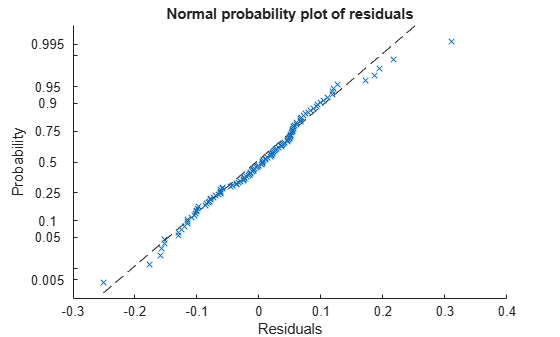plotResiduals

Class: LinearMixedModel

Plot residuals of linear mixed-effects model

Description

example

plotResiduals(lme,plottype) plots the raw conditional residuals of the linear mixed-effects model lme in a plot of the type specified by plottype.

example

plotResiduals(lme,plottype,Name,Value) also plots the residuals of the linear mixed-effects model lme with additional options specified by one or more name-value pair arguments. For example, you can specify the residual type to plot.

plotResiduals also accepts some other name-value pair arguments that specify the properties of the primary line in the plot. For those name-value pairs, see plot.

h = plotResiduals(___) returns a handle, h, to the lines or patches in the plot of residuals.

Input Arguments

expand all

Linear mixed-effects model, specified as a LinearMixedModel object constructed using fitlme or fitlmematrix.

Type of residual plot, specified as one of the following.

 'histogram' Default. Histogram of residuals 'caseorder' Residuals versus case (row) order 'fitted' Residuals versus fitted values 'lagged' Residuals versus lagged residual (r(t) versus r(t – 1)) 'probability' Normal probability plot 'symmetry' Symmetry plot

Example: plotResiduals(lme,'lagged')

Name-Value Arguments

Specify optional comma-separated pairs of Name,Value arguments. Name is the argument name and Value is the corresponding value. Name must appear inside quotes. You can specify several name and value pair arguments in any order as Name1,Value1,...,NameN,ValueN.

Residual type, specified by the comma-separated pair consisting of ResidualType and one of the following.

Residual TypeConditionalMarginal
'Raw'

${r}_{i}^{C}={\left[y-X\stackrel{^}{\beta }-Z\stackrel{^}{b}\right]}_{i}$

${r}_{i}^{M}={\left[y-X\stackrel{^}{\beta }\right]}_{i}$

'Pearson'

$p{r}_{i}^{C}=\frac{{r}_{i}^{C}}{{\sqrt{\left[{\stackrel{^}{Var}}_{y,b}\left(y-X\beta -Zb\right)\right]}}_{ii}}$

$p{r}_{i}^{M}=\frac{{r}_{i}^{M}}{\sqrt{{\left[{\stackrel{^}{Var}}_{y}\left(y-X\beta \right)\right]}_{ii}}}$

'Standardized'

$s{t}_{i}^{C}=\frac{{r}_{i}^{C}}{\sqrt{{\left[{\stackrel{^}{Var}}_{y}\left({r}^{C}\right)\right]}_{ii}}}$

$s{t}_{i}^{M}=\frac{{r}_{i}^{M}}{\sqrt{{\left[{\stackrel{^}{Var}}_{y}\left({r}^{M}\right)\right]}_{ii}}}$

Example: 'ResidualType','Standardized'

Output Arguments

expand all

Handle to the residual plot, returned as a handle.

Examples

expand all

weight contains data from a longitudinal study, where 20 subjects are randomly assigned to 4 exercise programs, and their weight loss is recorded over six 2-week time periods. This is simulated data.

Store the data in a table. Define Subject and Program as categorical variables.

tbl = table(InitialWeight,Program,Subject,Week,y);
tbl.Subject = categorical(tbl.Subject);
tbl.Program = categorical(tbl.Program);

Fit a linear mixed-effects model where the initial weight, type of program, week, and the interaction between the week and type of program are the fixed effects. The intercept and week vary by subject.

lme = fitlme(tbl,'y ~ InitialWeight + Program*Week + (Week|Subject)');

Plot the histogram of the raw residuals.

plotResiduals(lme)Plot the residuals versus the fitted values.

plotResiduals(lme,'fitted')There is no obvious pattern, so there are no immediate signs of heteroscedasticity.

Create the normal probability plot of residuals.

plotResiduals(lme,'probability')Data appears to be normal.

Find the observation number for the data that appears to be an outlier to the right of the plot.

find(residuals(lme)>0.25)
ans = 101

Create a box plot of the raw, Pearson, and standardized residuals.

r = residuals(lme);
pr = residuals(lme,'ResidualType','Pearson');
st = residuals(lme,'ResidualType','Standardized');
X = [r pr st];
boxplot(X,'labels',{'Raw','Pearson','Standardized'})All three box plots point out the outlier on the right tail of the distribution. The box plots of raw and Pearson residuals also point out a second possible outlier on the left tail. Find the corresponding observation number.

find(pr<-2)
ans = 10

Plot the raw residuals versus lagged residuals.

plotResiduals(lme,'lagged')There is no obvious pattern in the graph. The residuals do not appear to be correlated.